# Beyond Regression: Applying Machine Learning to Parametrics

Cost estimating has relied primarily upon regression analysis for parametric estimating. However, regression analysis is only one of many tools in data science and machine learning and is a small subset of supervised machine learning methods. In this paper we look at a variety of methods for predictive analysis for cost estimating, including other supervised methods such as neural networks, deep learning, and regression trees, as well as unsupervised methods and reinforcement learning.

Introduction

Abraham Maslow famously wrote in his classic book The Psychology of Science, “I suppose it is tempting, if the only tool you have is a hammer, to treat everything as if it were a nail.”

Cost estimating has relied primarily upon regression analysis for parametric estimating. However, regression analysis is only one of many tools in data science and machine learning. Indeed, traditional linear and nonlinear regression is a small subset of supervised machine learning methods. In this paper, we look at a variety of methods for predictive analysis for cost estimating, including other supervised methods such as neural networks, deep learning, and regression trees, as well as unsupervised methods and reinforcement learning.

We provide pros and cons of alternatives to regression, and a cross-sectional example that illustrates the similarities and differences of a variety of techniques outside of the traditional methodology.

Regression Analysis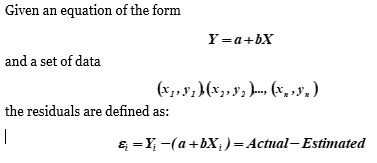This is also referred to as the “error” term since it is the difference between the actual cost and the estimated cost. Residuals or “errors” are an important consideration in modeling since they often drive the methods used for parameter calculation. For example, linear regression finds the “best fit” by finding the parameters a and b that minimize the sum of the squares of the residuals.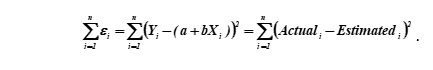This method was first developed by the mathematicians Legendre and Gauss in the early 19th century, who used it to predict the orbits of heavenly bodies using observed data. Francis Galton later applied this technique to find linear predictive relationships between various phenomena, such as the relationship between the heights of fathers and sons. Galton found a positive correlation between these heights but found a tendency to return or “regress” toward the average height, hence the term “regression analysis.”

In the spacecraft and defense industry it is more common to see nonlinear relationships between cost and cost drivers, e.g., Y = aXb. We are not going into details on this topic, but in the remainder of this presentation we will use the term regression to include the nonlinear case (see Smart 2017 for more details).

Beyond Regression

While regression analysis is a traditional tool that has been in use since the early 19th century, there are many other tools. A recent hot topic that covers most of these techniques is the realm of data science. The specific techniques that rival regression are part of machine learning. Computer scientists have used the powerful lever of computing power to make significant contributions to a variety of fields, including statistics and economics. There is a category of machine learning called reinforcement learning that is truly computer science and not statistics, but as far as it concerns us, machine learning is just a fancy word for statistics. Figure 1 displays the relationship of these topics among each other.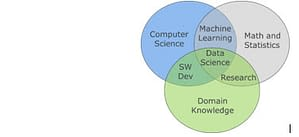Figure 1: Relationship of Machine Learning and Data Science to Computer Science, Mathematics, and Domain Knowledge

In the Venn Diagram that is Figure 1, you can see that machine learning is the intersection of statistics and computer science. Data science is the intersection of those two with “domain knowledge,” which is specific expertise in a specific field, e.g., epidemiology, and cost estimating! Yes, technically cost estimators practice data science. The distinction is largely the cost estimators do not typically work with “big data,” which refers to data that are “big” in at least one of volume, variety, or velocity. Big data typically is high volume, comes from a variety of sources, and is often available real-time. Cost estimators can use some of the same machine-learning techniques as those practicing “big data,” but we typically work in the realm of “small data” – a few data points collected (at best) on an annual basis.

There is a great deal of hyperbole, or hype, surrounding the topic of big data, about the potential for machine learning techniques to supplant traditional methods. Wired Magazine in 2008 trumpeted “The End of Theory” in an article which claimed that big data makes the scientific method obsolete. This reminds one of the authors of similar claims made in the 1990s about similar claims made about neural networks. While big data, data science, and machine learning have much to offer, the reality is not equal to the hype.

Before we can worry about “big data” we cost estimators need a much bigger volume of data. The initiative that is being spearheaded by the Office of the Secretary of Defense’s Cost Analysis and Program Evaluation organization on flexible cost reporting formats is a key step in this direction, but much more work remains to be done.

Also, if we focus solely on techniques without understanding the program, we risk making cost estimating a black art.

There are three categories of machine learning:

• Supervised learning – inputs and outputs are labelled
• Unsupervised learning – only the inputs are labelled
• Reinforcement learning – focus is on actions to maximize a goal function

In this paper we focus on supervised learning methods, which can be delineated into whether you are trying to learn a continuous or a discrete output. Cost is typically a continuous output, so we will focus on techniques to predict continuous outputs. As an aside, we should not completely overlook techniques that classify. As operations research analysts, we have skills to develop methods related to cost (e.g. success/failure) that are related to cost but do not directly predict cost. We have a larger skillset to aid leaders in making informed decisions, so we should look for opportunities to develop other types of tools and models.

These are the techniques we discuss in this paper:

• K-nearest neighbor
• Neural networks
• Regression trees
• Random forests
• Support vector machines
• Text analytics

We will discuss the pros and cons of each method and provide a cross-sectional worked example (except text analytics, which is outside the scope of this study).

K-Nearest Neighbor (KNN)

One of the simplest methods that can be used for regression is K-Nearest Neighbors (KNN).  The KNN algorithm is most commonly used for the classification of data, but it is also capable of predicting a numerical target based on a similarity measure.

KNN uses a ‘feature similarity’ to predict values of new data points.  The ‘nearest neighbors’ are chosen based on how closely the data points resemble provided inputs for the new data point.  Let’s look at a simple example as we walk through the steps to KNN. Table 1 presents the first 10 rows of a space dataset that has previously been normalized.  The bus is the infrastructure of the spacecraft, usually providing locations for space instruments. Data on the development costs for the bus are included and used as the dependent variable.  Independent variables of interest include bus weight, schedule (in log-transformed months), and design life (in log-transformed months) for the mission. The full dataset of 47 data points is used to estimate costs for a new space bus with the following inputs supplied:

• Bus Weight – 5.5
• Schedule – 3.8
• Design Life – 4.1

Table 1: KNN Bus Dataset

 Bus Cost Bus Weight Schedule Design Life Distance Rank 5.516401 5.960876 3.807393 4.094345 0.461 47 4.894587 4.906755 3.683933 3.295837 1.006 34 3.88881 4.406841 2.957169 3.178054 1.660 18 7.877092 7.662938 4.887575 4.430817 2.443 5 5.225369 6.244167 4.442651 3.091042 1.409 22 5.237665 5.772904 3.383498 4.204693 0.509 46 5.801111 6.551652 3.86073 4.553877 1.147 28 5.958923 6.687582 4.130947 4.564348 1.317 23 5.500074 5.722277 3.539242 3.178054 0.984 36 ? 5.5 3.8 4.1

First, we calculate the Euclidean distance for the continuous variables. The Euclidean distance is calculated as the square root of the sum of the squared differences between a new point (x) and an existing point (y).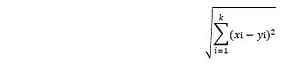If the variables are categorical, the Hamming distance should be used to measure the number of instances in which corresponding symbols are different in two strings of equal length.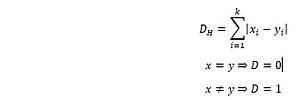If working with a dataset where variables have different measurement scales or there is a mixture of numerical and categorical variables, the variables should be standardized.  This will ensure that one variable does not have a higher influence on the distance than the other.

Once we have the distance of the new observation from the points in the dataset calculated, the next step is to choose the closest points, with the number of points to be considered defined as k.

K is the number of neighbors we will explore when we want to find the cost for a new bus. Determining the value of k is very important.  The results will change based on the value of k chosen.  For low values of k, the model tends to overfit the data, which leads to a high error rate.  On the other hand, high values of k can perform poorly as well.  The key is in determining the optimal value for k. This will depend entirely on the dataset being explored. A general rule of thumb is to set k equal to the square root of the data points.  Then, experiment by choosing +/- 1 of the starting k value.

Once k is determined, the bus costs from the k nearest data points (smallest distances) are averaged to estimate the cost of the new bus.

For this data, k is set to seven. The prediction for Bus Cost is equal to the average of Bus Cost for the top seven neighbors. For this example, Bus Cost is estimated to be \$750M.

The calculations for the missing Bus Cost using the dataset in Table 1 were done using Excel. Other software, like R, can be used to perform KNN. If using R, the target, or variable being estimated, must be a categorical or nominal variable.

One advantage of KNN technique is the method’s simplicity. It is a simple method to implement and understand the results. This method also provides predictions when conventional regression methods are unable to produce significant estimating relationships. A disadvantage is the frequency of overfitting data by choosing an improper value of k.

Neural Networks

Neural networks are the fundamental predictive set of algorithms in deep learning systems. First introduced in 1944 by Warren McCollough and Walter Pitts (University of Chicago), these algorithms are modeled loosely after the human brain and can be trained to recognize patterns that are numerical, contained in vectors, and are included in real-world data such as images, sound, text, and time series.

A neural net is composed of processing nodes that are interconnected. The nets are organized into layers of nodes and data moves through these nodes. Figure 2 presents the basic architecture of a neural net.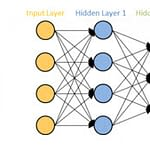Figure 2: Architecture of a Neural Net

The data comes into the input layer, is transformed in the hidden layers, and then scaled to the wanted outcome in the output layer. Each hidden layer, or node, receives the output from the previous node to which it is connected. The node is where computation occurs. These are multiplied by weights and summed. The sum is then transformed with a function and passed on to the nodes of the next layer or output as a result. The network is trained by searching for the weights that produce the desired output. The three key functions of neural networks are simplified as 1. Scoring input, 2. Calculating error, and 3. Adjusting Weights. As input enters the network, weights score the input and map it to a set of guesses the network makes at the end. The neural then takes a guess and compares the result to the data. Then, the network measurers error and adjust weights to the extent they contributed to the error. The algorithm repeats these steps until the error is minimized for the output.

The number of node layers through which the data passes determines the complexity of the analysis.  The deeper the network, the more complex the features the nodes recognize. This flexibility to enable neural networks to be capable of handling large and high dimensional datasets with numerous parameters and of nonlinear nature.

A function f(x) = y between any input, x, and output, y, is determined based on the neural network learning how x and y are related by understanding training datasets. Neural networks group unstructured data according to similarities within the inputs and classify data based on the labeled datasets used to train the predictive engine.

Using the same inputs as with the KNN method, calculating a prediction for Bus Cost using neural networks yields a Bus Cost of \$963M. Table 2 presents the neural network output obtained using the Bus Cost dataset.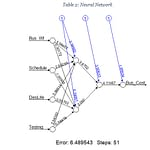An advantage of neural networks is the ability to learn and model complex, non-linear relationships.  Data for space systems and vehicle weapon systems have been known to be non-linear. These methods can be useful when trying to understand relationships for these commodities. Also, there are no restrictions on the input variables or assumptions on their distributions that have to be taken into consideration before the algorithm can be applied. An important disadvantage to consider is that neural networks do not explain how they converge on a solution. This can cause mistrust in the network.

Regression Trees

Another supervised learning technique is the tree-based learning algorithm, or regression trees. Tree-based methods are perfect for the visual learner since the data are split into homogenous groups, and the graphs present these splits with the use of branches (called decision nodes) and leaves (terminal nodes). The goal of tree-based methods is to partition data into smaller regions where interactions are manageable. They are useful when there is a non-linear and complex relationship between dependent and independent variables.

There are two types of trees: classification and regression trees.  This paper will focus on regression trees.  Regression trees are used when the dependent variable of interest is continuous. Figure 3 presents the components of a regression tree.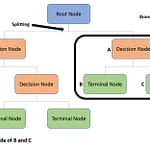Figure 3: Regression Tree Layout

The root node represents the entire population, or most commonly, the sample dataset that is being explored.  The root node splits into two or more decision nodes. The decision nodes represent the first set of homogenous groups discovered within the dataset. When a decision node can split no further, the branch ends in a leaf, or terminal node. Leaves represent a cell of partition and has a simple model for that cell; the model is the sample mean of the dependent variable.

Decision trees recursively split a dataset into partitions based on a criterion. Starting with the root node of the tree, the method asks a sequence of yes and no questions to determine the decision nodes.  The root node is split based on the most important predictor. Each split is labeled with the question and the branches between them are labeled with the answers.  The traditional approach is to split the set into two by choosing the split that minimizes uncertainty or entropy.

Regression tree analysis can be performed in R. The algorithm will choose the factor that minimizes the squared error. In the dataset used for this analysis, Bus Weight proved to be the most important factor. Figure 4 presents the regression tree produced from the Bus Cost dataset.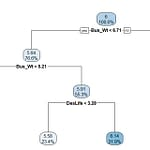Figure 4: GF Regression Tree

Bus Weight best minimizes the squared error when estimating development costs. There are three decision nodes that ask questions about the value of Bus Weight. Based on the value of Bus Weight, we end at one of the averages at the terminal nodes of the tree.  For example, if Bus Weight is 5.5 and Design Life is 4.1 (60 months in linear space), we follow the branches to determine the estimated development costs for a spacecraft bus should be \$464M.

There are several advantages to using regression trees. Visually, they are very easy to understand. They can be presented graphically and interpreted easily by non-technical reviewers. Regression trees can be used in preliminary data exploration to understand the most significant variables within a dataset.  Pairwise analysis combined with regression trees can help shorten the time running regression models in search of significant relationships. Regression trees can handle both numerical and categorical variables. Also, since this method is nonparametric, it does not rely on data belonging to a particular type of distribution.

There are disadvantages to regression trees. As with KNN, overfitting is also a common issue.  To remedy this, constraints must be defined on tree size which can be set in R and Python. Another disadvantage is despite the methods ability to handle both numerical and categorical variables, decision trees can lose information when it categorizes continuous variables in different categories.

Random Forests

One of the flaws of decision trees is that they tend to overfit the data leading to a confusion of noise with signal. To correct for this, an ensemble approach is used to create a random forest. This approach combines the estimates of multiple trees to produce an average. Random forests have been proven to provide better prediction (“wisdom of the crowd” effect). They are also more stable (robust to small amounts of noise). However, since the predictions are rather complex, there is no single equation or CER, which may make communicating the model to management challenging.

We applied to our data set – used 500 trees. We used random sampling of the dataset to randomly generate subsets of trees (bootstrap). Final prediction is an average of the result of 500 individual trees (bootstrap + aggregating = bagging).

Out-of-sample testing indicates that the mean squared error is 38% and the model explains 58% of the variation in the data. Using our example, the estimate is \$369 million.

Support Vector Machines

Support vector machines originated in the 1990s in the field of optical character recognition, where it was very successful. The basic idea for classification is to maximize the margin between classes, which yields maximally robust classification. To apply to continuous output, the analogous idea is to find an equation that is:

• As “flat” as possible, i.e., the coefficients are as small as possible
• Emphasis on sparseness, parsimony
• Makes model less sensitive to errors in inputs
• Minimizes the residuals that are outside a specified range of the estimate (-insensitive), e.g., 15%

For a linear equation Y = a+bX, with n data points the problem becomes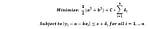where the delta values are non-negative, and the loss function is insensitive to residuals less than  (user specified), and a weight equal to C is given to the errors (controls for degree of parsimony). For example of insensitive losses, for a \$10 million project, you may not care about the residual as long as it is no larger than \$1 million.

Given a nonlinear equation Y = aXb, take log transforms of the data and apply the linear support vector set up. The insensitivity is now in log-space – the log of the differences between the actual and the estimate.

As an alternative to logarithmic transformation, you can apply the same notion to the absolute value of percentage difference between the actuals and the estimates, i.e.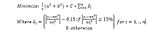For solving this optimization problem, can use Excel’s Solver capability. We use this latter formulation to apply to our example problem. We set the -insensitivity to 15% and C to a low value to emphasize parsimonious models.

The CER is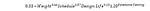The Pearson’s R2 is equal to 73%. For the 47 data points, the equation is within 15% of the actuals for 17 (36% of the total number of data points), and for the other 30 data points, the average error is 53%. The estimated Bus Cost is \$467M.

Text Analytics

Text analytics, or text mining, is supervised learning method that explores large quantities of textual data and finds patterns. Imagine having the capability of reading hundreds of pages of information in seconds to obtain information about one important topic. Text analytics provides this capability.

Text analytics provides the ability to process large amounts of structured or unstructured data from different mediums and output data related to a specific topic. This method seeks to find correlations between multiple documents, groups of words and single words. The text analytic tools search and scan data from documents, websites, databases and other data repositories and scans the data providing analysts the ability to search and explore relationships. The main goal is to break down large quantities of data into smaller, more manageable chunks to facilitate analysis.

Advantages of text analytics are obvious.  Having the ability to scan and dissect large amounts of data quickly is invaluable to analysts.  This method not only can find information on a specified topic but can also understand nuances in language. A disadvantage is that emotion cannot be captured during the review of text with this method. Without understanding tone and intent, sometimes text can be misinterpreted and information misused.

For this paper, text analytics is being introduced but not explored using data.

Conclusion

Traditional regression analysis is a tried-and-true method for cost estimating. The promise of big data and machine learning brings additional methods worth considering, including k-nearest neighbor, neural networks, regression trees, random forests, and support vector machines.

K-nearest neighbors is truly a non-parametric method. It is worth trying if you cannot find meaningful parametric relationships. The authors have found that this method came in very handy on a recent project.

Neural network is an artificial intelligence technique that has been successfully used in cost estimating in the past (see the references for examples). It is prone to overfitting, especially for small data sets. It was a big buzzword in the 1990s and is the basis for deep learning, a current hot topic in data science. However, before we can use deep learning in cost estimating, we need much bigger data sets.

Regression trees provides a different look at the data, in a tree format, which is useful in and of itself. However, it is prone to overfitting, and the results can be nonintuitive.

Random forests bring robustness and stability to the regression tree methodology. However, this method is largely a black box, and is there is no single tree or equation that produces the estimate. This can make the communication of this method to management a challenge.

Support vector machines are the closest in form to traditional regression and bring to it concepts that make regression more robust and less prone to error. The method is easy to implement in a spreadsheet using Excel Solver.

When using the same inputs, the methods produced the following Bus Costs:

• KNN – \$750M
• Neural Networks – \$963M
• Regression Trees – \$464M
• Random Forests – \$369M
• Support Vector Machines – \$467M

The methods produce results that range from \$369M to \$963M. It is interesting to see how, when using the same dataset, the different methods produce results that vary drastically. It is worth exploring further why the Neural Networks algorithm predicted costs must higher than the other methods.

R code used for the methods in this paper is available upon request.

By Kimberly Roye, CCEA, Christian Smart, Ph.D., CCEA of Galorath Federal, Inc.

References

1. Anderson, C., “The End of Theory: The Data Deluge Makes the Scientific Method Obsolete,” Wired, June 2008.
2. Davenport, D.H., and D.J. Patil, “Data Scientist: The Sexiest Job of the 21st Century,” Harvard Business Review, October 2012
3. Dean,E., “Neural Network Cost Estimating Relationships,” Proceedings of the 2010 ISPA-SCEA Conference, San Diego.
4. Hutchings, C., “An Approach Towards Determining Value Through the Application of Machine Learning,” Proceedings of the 2018 ICEAA Conference
5. Kaluzny, B.L, “An Application of Data Mining Algorithms for Shipbuilding Cost Estimation,” Proceedings of the 2011 ISPA-SCEA Conference
6. Mourikas, K., J. King, and D. Nelson, “Machine Learning Approach to Cost Analysis,” Proceedings of the 2017 ICEAA Conference.
7. Newell, A. and P.S. Rosembloom, “Mechanisms of Skill Acquisition and the Law of Practice,” in R Anderson(Ed.), Cognitive Skills and Their Acquisition, Hillsdale, NJ, Erlbaum.
8. Pincus, J., and O. Akbik, “Social Media and Submarines: How Machine Learning and Unconventional Methods Can Change Cost Estimating,” Proceedings of the 2018 ICEAA Conference.
9. Rao, Venky, “Introduction to Classification & Regression Trees”, January 2013
10. Singh,Aishwarya, “A Practical Introduction to K-Nearest Neighbors Algorithm for Regression”, August 2018.
Go Back

### Related Resources

#### Live Training: Effective Ways to Realistically Achieve Savings

Zoom Webinar: Thursday, October 28 @ 10 am PT / 1 pm ET Video will be made available to registrants … Read More Live Training: Effective Ways to Realistically Achieve Savings